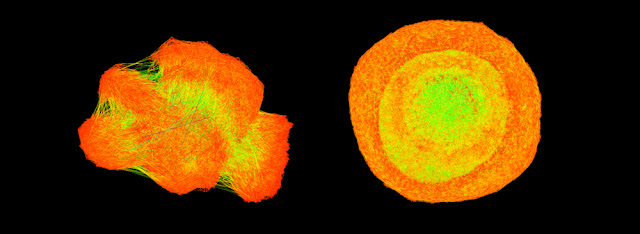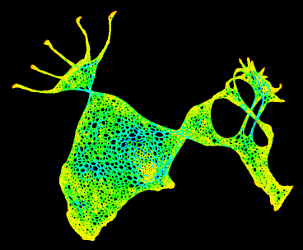## Saturday, March 5, 2011

### Digital Flowers and Data Protozoa in Zero Eden

Below:
a curated selection of graph drawings from the University of Florida Sparse Matrix Collection and a general-audience synopsis of what they are/how they were made.

a link to download the software used, if you'd like to try this at home.

In image links:
information on the specific properties of the individual matrices.Visualizing Sparse Matrices

by Tim Davis and Yifan Hu
(reposted from UFL website)

Scientific problems often lead to a system of equations to solve. One of us (Tim Davis) is an expert in methods for solving them, and he also collects equations from scientists with real problems to solve.

The pictures you see here are diagrams of some of those equations, created by Davis' colleague (Yifan Hu) who is an expert in visualizing them. Each dot, or intersection of two or more lines, is an equation for a particular unknown. A line is drawn between two equations if they share an unknown.

For example: (x): x+y=3, (y): x-y+z=0, (z): y+z=5 could be drawn as this picture:

x--y--z.
Not every equation involves every unknown. If it did, you would see this picture instead:

x---y
\ /
z
You've seen these kinds of figures before -- anytime you've looked an airline route map at the back of the magazine on an airplane. They're called graphs, as in "graph theory," with nodes (the dots) and edges (the lines between the dots).

To draw the dots and lines, we need to put the dots in place. But where?

Sometimes equations have a natural place. Take a helicopter and color it with dots, draw lines between the dots, and then remove the helicopter. You're left with just lines and dots, and the equations you've drawn might represent how the helicopter flies, or how it reacts to forces applied to it, or how it vibrates. Whatever they represent, the dots have a natural place in 3D space, so we can draw them there.What if you forgot it was a helicopter, and all you had were the equations? Or what if your equations did not represent a physical object, but something intangible such as a financial portfolio optimization problem, in which the unknowns and equations don't have a place? If you drew a diagram of investment decisions, "where" is an asset? "Where" is a decision? "Where" is an equation? Anywhere, and nowhere, but to draw it we need to put it somewhere.
We use physics to solve this problem. Make each line a spring, and give each dot an electrical charge. Then let the diagram "wobble" around so that the energy of this physical system dissipates, and the system reaches a stable minimal energy position. The final positions of the nodes now gives us the "where". This gives a good way to draw the graph. The lines between the nodes are given a color based on their length: hot (red) colors for short ones, green for middle ones, and blue (cool) for long ones.
You can see an animated demo of the dots and lines moving into place here: http://www.cise.ufl.edu/research/sparse/matrices

If you do this to the equations for the helicopter, you'll find that the graph looks like a warped version of the helicopter, below. The five main rotors are to the top left, the nose to the bottom left, and the tail rotor to the right.If you do this for a more abstract system of equations, you'll get something that shows how the equations relate to each other.
And quite often, the pictures are simply beautiful.

For the full gallery, please visit: http://www2.research.att.com/~yifanhu/GALLERY/GRAPHS/index.html
For the full paper, visit: http://www.cise.ufl.edu/~davis/techreports/matrices.pdf